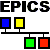## Experimental Physics and Industrial Control System

 <== Date ==> <== Thread ==> 1994  1995  1996  1997  1998  1999  2000  2001  2002  2003  2004  2005  2006  2007  2008  2009  2010  2011  2012  2013  2014  <2015>  2016  2017  2018  2019  2020 Index 1994  1995  1996  1997  1998  1999  2000  2001  2002  2003  2004  2005  2006  2007  2008  2009  2010  2011  2012  2013  2014  <2015>  2016  2017  2018  2019  2020

 Subject: calcPerform bit manipulation bug and fix From: Dirk Zimoch To: EPICS Date: Thu, 29 Oct 2015 15:14:06 +0100
```Hi all,

```
Bit manipulations in a calc or calcout record do not handle bit 31 correctly.
```
On my 64 bit Linux PC with EPICS 3.14.12, they calculate:

0xaaaaaaaa AND 0xffff0000 = 0x80000000 (wrong)
0xaaaaaaaa OR  0xffff0000 = 0xffffaaaa (OK)
0xaaaaaaaa XOR 0xffff0000 = 0x80000000 (wrong)
~0xaaaaaaaa               = 0x55555555 (OK)
~~0xaaaaaaaa              = 0xaaaaaaaa (OK)
0xaaaaaaaa >> 8           = 0xff800000 (wrong)
0xaaaaaaaa << 8           = 0x80000000 (wrong)

On my 32 bit Linux PC as well as on Windows 32 or 64 bit
and with EPICS 3.15.2 also on 64 bit Linux I get:

0xaaaaaaaa AND 0xffff0000 = 0x80000000 (wrong)
0xaaaaaaaa OR  0xffff0000 = 0x80000000 (wrong)
0xaaaaaaaa XOR 0xffff0000 = 0x80000000 (wrong)
~0xaaaaaaaa               = 0x7fffffff (wrong)
~~0xaaaaaaaa              = 0x80000000 (wrong)
0xaaaaaaaa >> 8           = 0xff800000 (wrong)
0xaaaaaaaa << 8           = 0x00000000 (wrong)

And on my MV5100 (PPC 32 bit) vxWorks with EPICS 3.14.12 I get:

0xaaaaaaaa AND 0xffff0000 = 0x7fffffff (wrong)
0xaaaaaaaa OR  0xffff0000 = 0x7fffffff (wrong)
0xaaaaaaaa XOR 0xffff0000 = 0x00000000 (wrong)
~0xaaaaaaaa               = 0x80000000 (wrong)
~~0xaaaaaaaa              = 0x7fffffff (wrong)
0xaaaaaaaa >> 8           = 0x007fffff (wrong)
0xaaaaaaaa << 8           = 0xffffff00 (wrong)

```
(Of course when printing the values on 64 bit systems, the values will be sign extended.)
```
```
So all are wrong and even differently wrong. (Maybe someone may add results for different architectures?)
```
Here is my test setup:

record (calcout, "DZ:CALC")
{
field(INPA, "0xaaaaaaaa")
field(INPB, "0xffff0000")
field(CALC, "A AND B")
field(PINI, "YES")
field(OUT,  "DZ:LO PP")
field(FLNK, "DZ:MBBI")
}

record (longout, "DZ:LO")

record (mbbiDirect, "DZ:MBBI")
{
field (DTYP, "Raw Soft Channel")
field (INP,  "DZ:CALC")
field (SHFT, "16")
}

```
The reason for the wrong behavior is that conversions from floating point to integer are "undefined" if the value does not fit.
```
```
For the calc record 0xaaaaaaaa is a positive double value (2863311530.0). But during the bit calculation, it is converted to a signed integer, long in 3.14, epicsInt32 in 3.15. Since the value is too large, a correct conversion is impossible and compiler and CPU may do anything, for example set the value to 0x80000000 or 0x7fffffff.
```
K&R:
A6.3 Integer and Floating
```
When a value of floating type is converted to integral type, the fractional part is discarded; if the resulting value cannot be represented in the integral type, the behavior is undefined. In particular, the result of converting negative floating values to unsigned integral types is not specified.
```
```
BTW: "the behavior is undefined" may also legally mean: "abort the program" or "freeze the CPU".
```
```
So we have two problems here with bit patterns using bit 31. Either they are represented as a large positive floating point number which cannot be converted to a signed 32 bit integer or they are represented as a negative floating point number which cannot be converted to an unsigned integer. Luckily it turns out that the second case seems not to be a problem on any architecture I tried, even though it "not specified" according to K&R.
```
The fix is:
* Before the conversion, cast double value to unsigned integer
* After the conversion, cast to signed integer and then write to double
```
* For right shift cast double to unsigned first, then to signed, then shift and last write back the result to double. (This produces sign extended right shift.)
```
case BIT_OR:
itop = (epicsUInt32) *ptop--;
*ptop = (epicsInt32) ((epicsUInt32) *ptop | itop);
break;

case BIT_AND:
itop = (epicsUInt32) *ptop--;
*ptop = (epicsInt32) ((epicsUInt32) *ptop & itop);
break;

case BIT_EXCL_OR:
itop = (epicsUInt32) *ptop--;
*ptop = (epicsInt32) ((epicsUInt32) *ptop ^ itop);
break;

case BIT_NOT:
itop = (epicsUInt32) *ptop;
*ptop = ~itop;
break;

case RIGHT_SHIFT:
itop = (epicsInt32) *ptop--;
*ptop = (epicsInt32) (epicsUInt32) *ptop >> itop;
break;

case LEFT_SHIFT:
itop = (epicsInt32) *ptop--;
*ptop = (epicsInt32) ((epicsUInt32) *ptop << itop);
break;

After the fix I get what I expect:
0xaaaaaaaa AND 0xffff0000 = 0xaaaa0000
0xaaaaaaaa OR  0xffff0000 = 0xffffaaaa
0xaaaaaaaa XOR 0xffff0000 = 0x5555aaaa
~0xaaaaaaaa               = 0x55555555
~~0xaaaaaaaa              = 0xaaaaaaaa
0xaaaaaaaa >> 8           = 0xffaaaaaa
0xaaaaaaaa << 8           = 0xaaaaaa00

```
Tested on vxWorks PPC 32 bit, Linux PC 64 and 32 bit, Linux ARM6 LE 32 bit (all gcc).
```
Can someone test other architectures/compilers?

```
There is still the general problem of writing double values to integer fields through a link. This needs to fixed somewhere else in EPICS.
```

Dirk
```

Navigate by Date:
Prev: The EPICS Archiver Appliance has moved to GitHub. Shankar, Murali
Next: RE: Unable to build support modules on Windows, with base 3.15.2 Heesterman, Peter J
Index: 1994  1995  1996  1997  1998  1999  2000  2001  2002  2003  2004  2005  2006  2007  2008  2009  2010  2011  2012  2013  2014  <20152016  2017  2018  2019  2020